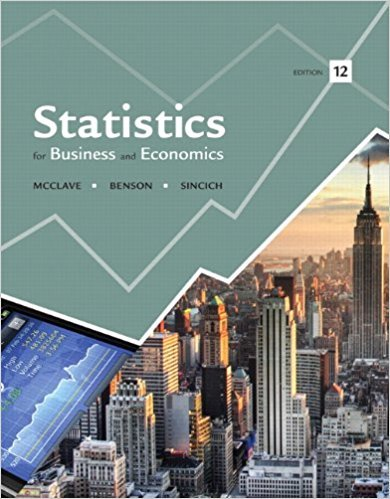×
Get Full Access to Statistics For Business And Economics - 12 Edition - Chapter 3 - Problem 48e
Get Full Access to Statistics For Business And Economics - 12 Edition - Chapter 3 - Problem 48e

×

# Galileo’s Passedix game. Passedix is a game of chanceISBN: 9780321826237 51

## Solution for problem 48E Chapter 3

Statistics for Business and Economics | 12th Edition

• Textbook Solutions
• 2901 Step-by-step solutions solved by professors and subject experts
• Get 24/7 help from StudySoup virtual teaching assistantsStatistics for Business and Economics | 12th Edition

4 5 1 256 Reviews
28
2
Problem 48E

Problem 48E

Galileo’s Passedix game. Passedix is a game of chance played with three fair dice. Players bet whether the sum of the faces shown on the dice will be above or below 10. During the late sixteenth century, the astronomer and mathematician Galileo Galilei was asked by the Grand Duke of Tuscany to explain why “the chance of throwing a total of 9 with three fair dice was less than that of throwing a total of 10” (Interstat, Jan. 2004). The grand duke believed that the chance should be the same because “there are an equal number of partitions of the numbers 9 and 10.” Find the flaw in the grand duke’s reasoning and answer the question posed to Galileo.

Step-by-Step Solution:

Step 1 of 1

We are asked to find the reason that the chance of throwing a total of 9 with three fair dice was less than that of throwing a total of 10.

The grand duke believed that the chance should be the same because “ there are an equal number of partitions of the number 9 and 10.”

We need to find the flaw in the grand duke’s reasoning and answer the right reasoning.

Since we have given the three fair dice, then there are 6 possible outcomes of each dice.

Hence the possible combinations in the sample space would beLets denote the first dice asthe second dice asand the Third dice asLets form the sample space of getting a total ofby three dice.Similarly, getting total of 10 by three dice,Since the grand duke believed there are an equal number of partitions of the number 9 and 10.

However, if we take into account the three identities (A, B, and C) of the dice, then there are various ways to get each partition.

For instance, to get a partition of 126, we will havecombinations like (126, 162, 216, 261, 612, 621).

Similarly,  we can think of a number of ways for each partition.

 The chance of throwing a total of 9 Number of ways 126 6 135 6 144 3 225 3 234 6 333 1 Total 25

Similarly, a number of ways of getting a total of 10 for each partition.

 The chance of throwing a total of 10 Number of ways 136 6 145 6 244 3 226 3 235 6 334 3 Total 27

Therefore, there is a total of 25 ways of getting a sum of 9 and 27 ways of getting a sum of 10.

Thus, the chance of throwing a total of 9 is less than the chance of throwing a total of 10.

Hence there is a flaw in the grand duke’s reasoning.

Step 2 of 1

##### ISBN: 9780321826237

The full step-by-step solution to problem: 48E from chapter: 3 was answered by , our top Business solution expert on 07/21/17, 05:42AM. Since the solution to 48E from 3 chapter was answered, more than 1148 students have viewed the full step-by-step answer. Statistics for Business and Economics was written by and is associated to the ISBN: 9780321826237. The answer to “Galileo’s Passedix game. Passedix is a game of chance played with three fair dice. Players bet whether the sum of the faces shown on the dice will be above or below 10. During the late sixteenth century, the astronomer and mathematician Galileo Galilei was asked by the Grand Duke of Tuscany to explain why “the chance of throwing a total of 9 with three fair dice was less than that of throwing a total of 10” (Interstat, Jan. 2004). The grand duke believed that the chance should be the same because “there are an equal number of partitions of the numbers 9 and 10.” Find the flaw in the grand duke’s reasoning and answer the question posed to Galileo.” is broken down into a number of easy to follow steps, and 119 words. This textbook survival guide was created for the textbook: Statistics for Business and Economics , edition: 12. This full solution covers the following key subjects: grand, dice, chance, duke, galileo. This expansive textbook survival guide covers 15 chapters, and 1631 solutions.

Unlock Textbook Solution